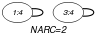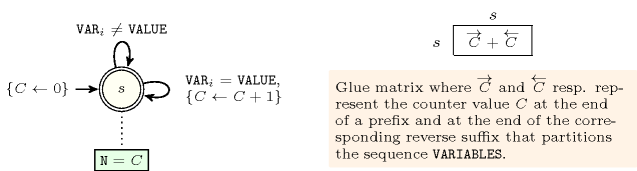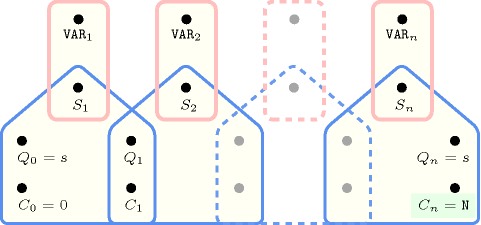## 5.155. exactly

Origin
Constraint

$\mathrm{𝚎𝚡𝚊𝚌𝚝𝚕𝚢}\left(𝙽,\mathrm{𝚅𝙰𝚁𝙸𝙰𝙱𝙻𝙴𝚂},\mathrm{𝚅𝙰𝙻𝚄𝙴}\right)$

Synonym

$\mathrm{𝚌𝚘𝚞𝚗𝚝}$.

Arguments
 $𝙽$ $\mathrm{𝚒𝚗𝚝}$ $\mathrm{𝚅𝙰𝚁𝙸𝙰𝙱𝙻𝙴𝚂}$ $\mathrm{𝚌𝚘𝚕𝚕𝚎𝚌𝚝𝚒𝚘𝚗}\left(\mathrm{𝚟𝚊𝚛}-\mathrm{𝚍𝚟𝚊𝚛}\right)$ $\mathrm{𝚅𝙰𝙻𝚄𝙴}$ $\mathrm{𝚒𝚗𝚝}$
Restrictions
 $𝙽\ge 0$ $𝙽\le |\mathrm{𝚅𝙰𝚁𝙸𝙰𝙱𝙻𝙴𝚂}|$ $\mathrm{𝚛𝚎𝚚𝚞𝚒𝚛𝚎𝚍}$$\left(\mathrm{𝚅𝙰𝚁𝙸𝙰𝙱𝙻𝙴𝚂},\mathrm{𝚟𝚊𝚛}\right)$
Purpose

Exactly $𝙽$ variables of the $\mathrm{𝚅𝙰𝚁𝙸𝙰𝙱𝙻𝙴𝚂}$ collection are assigned value $\mathrm{𝚅𝙰𝙻𝚄𝙴}$.

Example
$\left(2,〈4,2,4,5〉,4\right)$

The $\mathrm{𝚎𝚡𝚊𝚌𝚝𝚕𝚢}$ constraint holds since exactly $𝙽=2$ variables of the $\mathrm{𝚅𝙰𝚁𝙸𝙰𝙱𝙻𝙴𝚂}=〈4,2,4,5〉$ collection are assigned value $\mathrm{𝚅𝙰𝙻𝚄𝙴}=4$.

Typical
 $𝙽>0$ $𝙽<|\mathrm{𝚅𝙰𝚁𝙸𝙰𝙱𝙻𝙴𝚂}|$ $|\mathrm{𝚅𝙰𝚁𝙸𝙰𝙱𝙻𝙴𝚂}|>1$
Symmetries
• Items of $\mathrm{𝚅𝙰𝚁𝙸𝙰𝙱𝙻𝙴𝚂}$ are permutable.

• An occurrence of a value of $\mathrm{𝚅𝙰𝚁𝙸𝙰𝙱𝙻𝙴𝚂}.\mathrm{𝚟𝚊𝚛}$ that is different from $\mathrm{𝚅𝙰𝙻𝚄𝙴}$ can be replaced by any other value that is also different from $\mathrm{𝚅𝙰𝙻𝚄𝙴}$.

Arg. properties
• Functional dependency: $𝙽$ determined by $\mathrm{𝚅𝙰𝚁𝙸𝙰𝙱𝙻𝙴𝚂}$ and $\mathrm{𝚅𝙰𝙻𝚄𝙴}$.

• Aggregate: $𝙽\left(+\right)$, $\mathrm{𝚅𝙰𝚁𝙸𝙰𝙱𝙻𝙴𝚂}\left(\mathrm{𝚞𝚗𝚒𝚘𝚗}\right)$, $\mathrm{𝚅𝙰𝙻𝚄𝙴}\left(\mathrm{𝚒𝚍}\right)$.

Systems

generalisation: $\mathrm{𝚊𝚖𝚘𝚗𝚐}$ ($\mathrm{𝚌𝚘𝚗𝚜𝚝𝚊𝚗𝚝}$ replaced by $\mathrm{𝚟𝚊𝚛𝚒𝚊𝚋𝚕𝚎}$ and $\mathrm{𝚟𝚊𝚕𝚞𝚎}$ replaced by $\mathrm{𝚕𝚒𝚜𝚝}$ of $\mathrm{𝚟𝚊𝚕𝚞𝚎𝚜}$).

implies: $\mathrm{𝚊𝚝𝚕𝚎𝚊𝚜𝚝}$ ($=𝙽$ replaced by $\ge 𝙽$), $\mathrm{𝚊𝚝𝚖𝚘𝚜𝚝}$ ($=𝙽$ replaced by $\le 𝙽$).

Keywords
Arc input(s)

$\mathrm{𝚅𝙰𝚁𝙸𝙰𝙱𝙻𝙴𝚂}$

Arc generator
$\mathrm{𝑆𝐸𝐿𝐹}$$↦\mathrm{𝚌𝚘𝚕𝚕𝚎𝚌𝚝𝚒𝚘𝚗}\left(\mathrm{𝚟𝚊𝚛𝚒𝚊𝚋𝚕𝚎𝚜}\right)$

Arc arity
Arc constraint(s)
$\mathrm{𝚟𝚊𝚛𝚒𝚊𝚋𝚕𝚎𝚜}.\mathrm{𝚟𝚊𝚛}=\mathrm{𝚅𝙰𝙻𝚄𝙴}$
Graph property(ies)
$\mathrm{𝐍𝐀𝐑𝐂}$$=𝙽$

Graph model

Since each arc constraint involves only one vertex ($\mathrm{𝚅𝙰𝙻𝚄𝙴}$ is fixed), we employ the $\mathrm{𝑆𝐸𝐿𝐹}$ arc generator in order to produce a graph with a single loop on each vertex.

Parts (A) and (B) of Figure 5.155.1 respectively show the initial and final graph associated with the Example slot. Since we use the $\mathrm{𝐍𝐀𝐑𝐂}$ graph property, the loops of the final graph are stressed in bold. The $\mathrm{𝚎𝚡𝚊𝚌𝚝𝚕𝚢}$ constraint holds since exactly two variables are assigned value 4.

##### Figure 5.155.1. Initial and final graph of the $\mathrm{𝚎𝚡𝚊𝚌𝚝𝚕𝚢}$ constraint(a) (b)
Automaton

Figure 5.155.2 depicts the automaton associated with the $\mathrm{𝚎𝚡𝚊𝚌𝚝𝚕𝚢}$ constraint. To each variable ${\mathrm{𝚅𝙰𝚁}}_{i}$ of the collection $\mathrm{𝚅𝙰𝚁𝙸𝙰𝙱𝙻𝙴𝚂}$ corresponds a 0-1 signature variable ${S}_{i}$. The following signature constraint links ${\mathrm{𝚅𝙰𝚁}}_{i}$ and ${S}_{i}$: ${\mathrm{𝚅𝙰𝚁}}_{i}=\mathrm{𝚅𝙰𝙻𝚄𝙴}⇔{S}_{i}$.

##### Figure 5.155.2. Automaton (with one counter) of the $\mathrm{𝚎𝚡𝚊𝚌𝚝𝚕𝚢}$ constraint and its glue matrix##### Figure 5.155.3. Hypergraph of the reformulation corresponding to the automaton (with one counter) of the $\mathrm{𝚎𝚡𝚊𝚌𝚝𝚕𝚢}$ constraint: since all states variables ${Q}_{0},{Q}_{1},\cdots ,{Q}_{n}$ are fixed to the unique state $s$ of the automaton, the transitions constraints share only the counter variable $C$ and the constraint network is Berge-acyclic